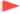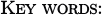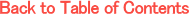Adv. Math. Econ. Volume 2, pp.87-118 (2000)

Fixed point theorems and the existence of economic equilibria based on conditions for local directions of mappings

Ken Urai
Graduate School of Economics, Osaka University, Machikaneyama, Toyonaka, Osaka 560-0043, JapanFixed point theorems for set valued mappings are reexamined from a unified viewpoint on local directions of the values of a mapping on a subset of a Hausdorff topological vector space to itself. Some basic fixed point theorems, such as Kakutani's and Browder's, are generalized so that we could apply them to game theoretic and economic equilibrium existence problems with non-ordered preferences having neither global continuity nor convexity conditions. Relations of our main results to other mathematical theorems such as Fan-Browder's theorem, maximal element existence theorem for L-majorized mappings, Eaves' theorem, KKM and KKMS theorem, are also studied.Kakutani's fixed point theorem, Browder's Rxed point theorem, General equilibrium, Excess demand, Non-ordered preference, Nash equilibrium, Abstract economy, Gale-Nikaido-Debreu theorem, L-majorized mappings, Eaves' Theorem, KKM Theorem, KKMS Theorem.